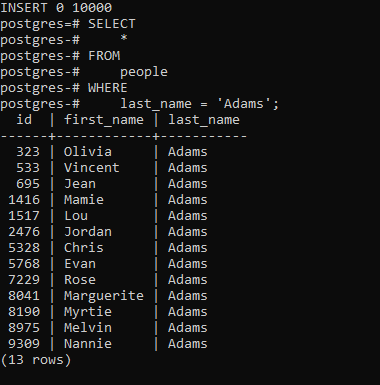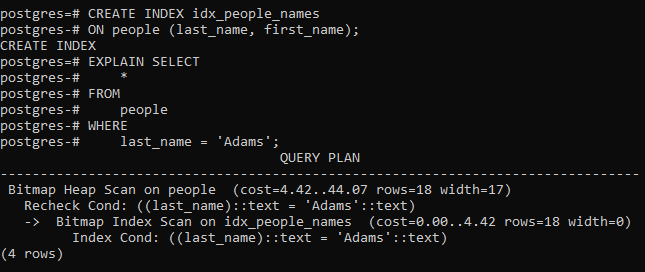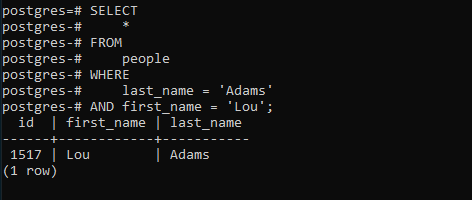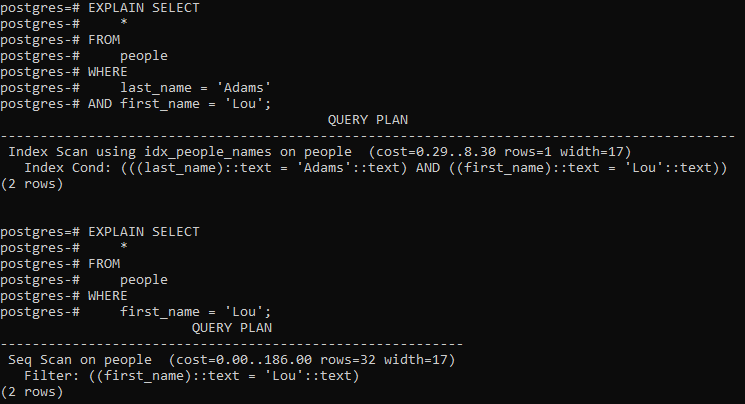# PostgreSQL – Multicolumn Indexes

• Last Updated : 28 Aug, 2020

In PostgreSQL, the multicolumn indexes are indexes defined on more than one column of a table.

You can create an index on more than one column of a table. This index is called a multicolumn index, a composite index, a combined index, or a concatenated index. A multicolumn index can have a maximum of 32 columns of a table. The limit can be changed by modifying the pg_config_manual.h when building PostgreSQL. In addition, only B-tree, GIST, GIN, and BRIN index types support multicolumn indexes.

The following syntax shows how to create a multicolumn index:

```Syntax:
CREATE INDEX index_name
ON table_name(a, b, c, ...);
```

When defining a multicolumn index, one should place the columns which are often used in the WHERE clause at the beginning of the column list and the columns that are less frequently used in the condition after.

In the above syntax, the PostgreSQL optimizer will consider using the index in the following cases:

```WHERE a = v1 and b = v2 and c = v3;
```

or,

```WHERE a = v1 and b = v2;
```

or,

```WHERE a = v1;
```

However, it will not consider using the index in the following cases:

```WHERE  c = v3;
```

or,

```WHERE b = v2 and c = v3;
```

Example:

To demonstrate multicolumn indexes, we will create a new table named people with three columns: id, first name, and last name:

```CREATE TABLE people(
id INT GENERATED BY DEFAULT AS IDENTITY,
first_name VARCHAR(50) NOT NULL,
last_name VARCHAR(50) NOT NULL
);
```

You can add data to the people through this file.

The following statement finds people whose last name is Adams:

```SELECT
*
FROM
people
WHERE
```

This will result in the following:As shown clearly in the output, PostgreSQL performed the sequential scan on the people table to find the corresponding rows because there was no index defined for the last_name column.

Let’s define a B-tree index on both last_name and first_name columns. Assuming that searching for people by the last name is more often than by the first name, we define the index with the following column order:

```CREATE INDEX idx_people_names
ON people (last_name, first_name);
```

Now, if you search for people whose last name is Adams, the PostgreSQL optimizer will use the index as shown in the output of the following statement:

```EXPLAIN SELECT
*
FROM
people
WHERE
```

This will output the below:The following statement finds the person whose last name is Adams and the first name is Lou:

```SELECT
*
FROM
people
WHERE
AND first_name = 'Lou';
```

This will lead to the following:The PostgreSQL Optimizer used the index for this statement because both columns in the WHERE clause are all in the index:

```EXPLAIN SELECT
*
FROM
people
WHERE
AND first_name = 'Lou';
```

However, if you search for people whose first name is Lou, PostgreSQL will perform sequential scan the table instead of using the index as shown in the output of the following statement:

```EXPLAIN SELECT
*
FROM
people
WHERE
first_name = 'Lou';
```

Output:My Personal Notes arrow_drop_up